# An Introduction to t_TOST

### A new function for TOST with t-tests

#### 2022-12-13

In an effort to make TOSTER more informative and easier to use, a new function t_TOST has been created. This function operates very similarly to base R’s t.test function, but performs 3 t-tests (one two-tailed and two one-tailed tests). In addition, this function has a generic method where two vectors can be supplied or a formula can be given (e.g.,y ~ group). This function also makes it easier to switch between types of t-tests. All three types (two sample, one sample, and paired samples) can be performed/calculated from the same function. Moreover, the summary information and visualizations have been upgraded. This should make the decisions derived from the function more informative and user-friendly.

Also, t_TOST is not limited to equivalence tests. Minimal effects testing (MET) is now possible. MET is useful for situations where the hypothesis is about a minimal effect and the null hypothesis is equivalence.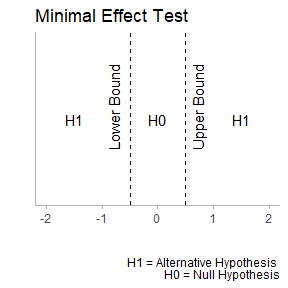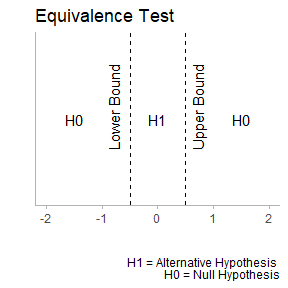In the general introduction to this package we detailed how to look at old results and how to apply TOST to interpreting those results. However, in many cases, users may have new data that needs to be analyzed. Therefore, t_TOST can be applied to new data. This vignette will use the iris and the sleep data.

data('sleep')
data('iris')

# Independent Groups

For this example, we will use the sleep data. In this data there is a group variable and an outcome extra.

head(sleep)
#>   extra group ID
#> 1   0.7     1  1
#> 2  -1.6     1  2
#> 3  -0.2     1  3
#> 4  -1.2     1  4
#> 5  -0.1     1  5
#> 6   3.4     1  6

We will assume the data are independent, and that we have equivalence bounds of +/- 0.5. All we need to do is provide the formula, data, and eqbound arguments for the function to run appropriately. In addition, we can set the var.equal argument (to assume equal variance), and the paired argument (sets if the data is paired or not). Both are logical indicators that can be set to TRUE or FALSE. The alpha is automatically set to 0.05 but this can also be adjusted by the user. The Hedges correction is also automatically calculated, but this can be overridden with the bias_correction argument. The hypothesis is automatically set to “EQU” for equivalence but if a minimal effect is of interest then “MET” can be supplied. Note: for this example, we will set smd_ci to “goulet” since it will reduce the time to produce plots.

res1 = t_TOST(formula = extra ~ group,
data = sleep,
eqb = .5,
smd_ci = "goulet")

res1a = t_TOST(x = subset(sleep,group==1)$extra, y = subset(sleep,group==2)$extra,
eqb = .5)

Once the function has run, we can print the results with the print command. This provides a verbose summary of the results.

print(res1)
#>
#> Welch Two Sample t-test
#>
#> The equivalence test was non-significant, t(17.78) = -1.272, p = 8.9e-01
#> The null hypothesis test was non-significant, t(17.78) = -1.861, p = 7.94e-02
#> NHST: don't reject null significance hypothesis that the effect is equal to zero
#> TOST: don't reject null equivalence hypothesis
#>
#> TOST Results
#>                 t    df p.value
#> t-test     -1.861 17.78   0.079
#> TOST Lower -1.272 17.78   0.890
#> TOST Upper -2.450 17.78   0.012
#>
#> Effect Sizes
#>                Estimate     SE               C.I. Conf. Level
#> Raw             -1.5800 0.8491 [-3.0534, -0.1066]         0.9
#> Hedges's g(av)  -0.7965 0.4976 [-1.6843, -0.0615]         0.9
#> Note: SMD confidence intervals are an approximation. See vignette("SMD_calcs").

Another nice feature is the generic plot method that can provide a visual summary of the results. All of the plots in this package were inspired by the concurve R package. There are two types of plots that can be produced. The first, and default, is the consonance density plot (type = "cd").

plot(res1, type = "cd")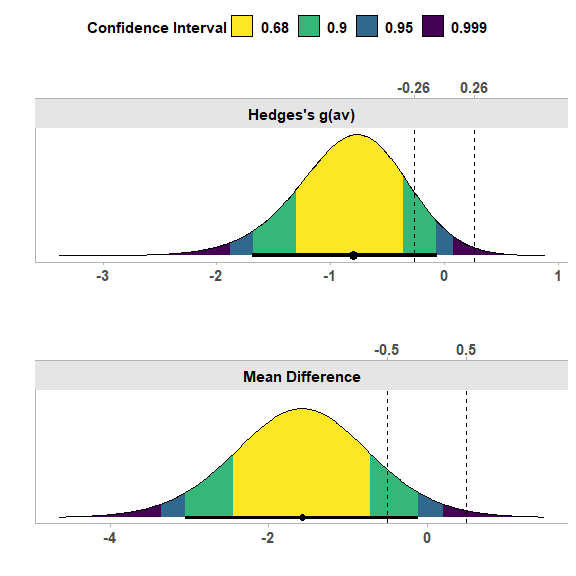The shading pattern can be modified with the ci_shades.

# Set to shade only the 90% and 95% CI areas
plot(res1, type = "cd",
ci_shades = c(.9,.95))

Consonance plots, where all confidence intervals can be simultaneous plotted, can also be produced. The advantage here is multiple confidence interval lines can plotted at once.

plot(res1, type = "c",
ci_lines =  c(.9,.95))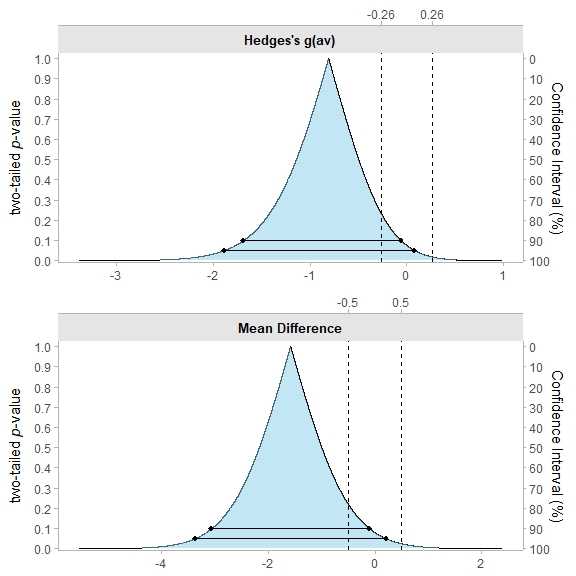# Paired Samples

To perform a paired samples TOST, the process does not change much. We could process the test the same way by providing a formula. All we would need to then is change paired to TRUE.

res2 = t_TOST(formula = extra ~ group,
data = sleep,
paired = TRUE,
eqb = .5)
res2
#>
#> Paired t-test
#>
#> The equivalence test was non-significant, t(9) = -2.777, p = 9.89e-01
#> The null hypothesis test was significant, t(9) = -4.062, p = 2.83e-03
#> NHST: reject null significance hypothesis that the effect is equal to zero
#> TOST: don't reject null equivalence hypothesis
#>
#> TOST Results
#>                 t df p.value
#> t-test     -4.062  9   0.003
#> TOST Lower -2.777  9   0.989
#> TOST Upper -5.348  9 < 0.001
#>
#> Effect Sizes
#>               Estimate    SE               C.I. Conf. Level
#> Raw             -1.580 0.389   [-2.293, -0.867]         0.9
#> Hedges's g(z)   -1.174 0.411 [-1.8046, -0.4977]         0.9
#> Note: SMD confidence intervals are an approximation. See vignette("SMD_calcs").

However, we may have two vectors of data that are paired. So instead we may want to just provide those separately rather than using a data set and setting the formula. This can be demonstrated with the “iris” data.

res3 = t_TOST(x = iris$Sepal.Length, y = iris$Sepal.Width,
paired = TRUE,
eqb = 1)
res3
#>
#> Paired t-test
#>
#> The equivalence test was non-significant, t(149) = 22.319, p = 1e+00
#> The null hypothesis test was significant, t(149) = 34.815, p = 1.85e-73
#> NHST: reject null significance hypothesis that the effect is equal to zero
#> TOST: don't reject null equivalence hypothesis
#>
#> TOST Results
#>                t  df p.value
#> t-test     34.82 149 < 0.001
#> TOST Lower 47.31 149 < 0.001
#> TOST Upper 22.32 149       1
#>
#> Effect Sizes
#>               Estimate      SE             C.I. Conf. Level
#> Raw              2.786 0.08002 [2.6536, 2.9184]         0.9
#> Hedges's g(z)    2.828 0.18257 [2.5252, 3.1244]         0.9
#> Note: SMD confidence intervals are an approximation. See vignette("SMD_calcs").

We may want to perform a Minimal Effect Test with the hypothesis argument set to “MET”.

res3a = t_TOST(x = iris$Sepal.Length, y = iris$Sepal.Width,
paired = TRUE,
hypothesis = "MET",
eqb = 1,
smd_ci = "goulet")
res3a
#>
#> Paired t-test
#>
#> The minimal effect test was significant, t(149) = 47.312, p = 1.13e-49
#> The null hypothesis test was significant, t(149) = 34.815, p = 1.85e-73
#> NHST: reject null significance hypothesis that the effect is equal to zero
#> TOST: reject null MET hypothesis
#>
#> TOST Results
#>                t  df p.value
#> t-test     34.82 149 < 0.001
#> TOST Lower 47.31 149       1
#> TOST Upper 22.32 149 < 0.001
#>
#> Effect Sizes
#>               Estimate      SE             C.I. Conf. Level
#> Raw              2.786 0.08002 [2.6536, 2.9184]         0.9
#> Hedges's g(z)    2.835 0.25311 [2.5719, 3.1284]         0.9
#> Note: SMD confidence intervals are an approximation. See vignette("SMD_calcs").

# One Sample t-test

In other cases we may just have a one sample test. If that is the case all we have to do is supply the x argument for the data. For this test we may hypothesis that the mean of Sepal.Length is not more than 5.5 points greater or less than 8.5.

res4 = t_TOST(x = iris$Sepal.Length, hypothesis = "EQU", eqb = c(5.5,8.5), smd_ci = "goulet") res4 #> #> One Sample t-test #> #> The equivalence test was significant, t(149) = 5.078, p = 5.62e-07 #> The null hypothesis test was significant, t(149) = 86.425, p = 3.33e-129 #> NHST: reject null significance hypothesis that the effect is equal to zero #> TOST: reject null equivalence hypothesis #> #> TOST Results #> t df p.value #> t-test 86.425 149 < 0.001 #> TOST Lower 5.078 149 < 0.001 #> TOST Upper -39.293 149 < 0.001 #> #> Effect Sizes #> Estimate SE C.I. Conf. Level #> Raw 5.843 0.06761 [5.7314, 5.9552] 0.9 #> Hedges's g 7.021 0.42002 [6.4067, 7.7882] 0.9 #> Note: SMD confidence intervals are an approximation. See vignette("SMD_calcs"). # Only have the summary statistics? No problem! In some cases you may only have access to the summary statistics. Therefore, we created a function, tsum_TOST,to perform the same tests just based on the summary statistics. This involves providing the function with a number of different arguments. • n1 & n2 the sample sizes (only n1 needs to be provided for one sample case) • m1 & m2 the sample means • sd1 & sd2 the sample standard deviation • r12 the correlation between each if paired is set to TRUE The results from above can be replicated with the tsum_TOST res_tsum = tsum_TOST( m1 = mean(iris$Sepal.Length, na.rm=TRUE),
sd1 = sd(iris$Sepal.Length, na.rm=TRUE), n1 = length(na.omit(iris$Sepal.Length)),
hypothesis = "EQU",
eqb = c(5.5,8.5)
)

res_tsum
#>
#> One-sample t-Test
#>
#> The equivalence test was significant, t(149) = 5.078, p = 5.62e-07
#> The null hypothesis test was significant, t(149) = 86.425, p = 3.33e-129
#> NHST: reject null significance hypothesis that the effect is equal to zero
#> TOST: reject null equivalence hypothesis
#>
#> TOST Results
#>                  t  df p.value
#> t-test      86.425 149 < 0.001
#> TOST Lower   5.078 149 < 0.001
#> TOST Upper -39.293 149 < 0.001
#>
#> Effect Sizes
#>            Estimate      SE             C.I. Conf. Level
#> Raw           5.843 0.06761 [5.7314, 5.9552]         0.9
#> Hedges's g    7.021 0.41350  [6.327, 7.6914]         0.9
#> Note: SMD confidence intervals are an approximation. See vignette("SMD_calcs").
plot(res_tsum)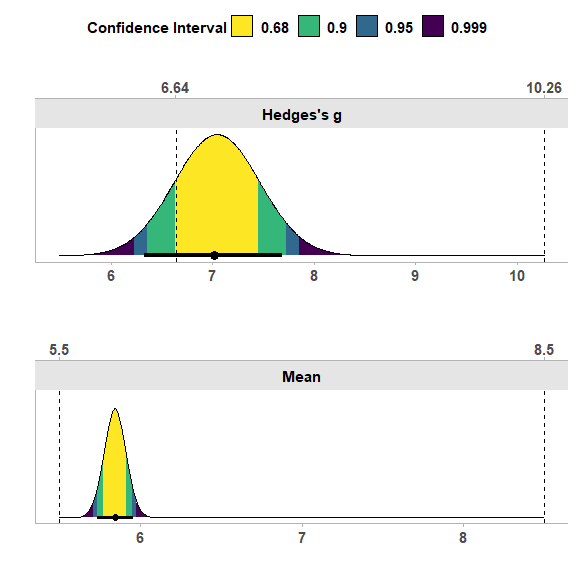# Power Analysis for t-test based TOST

A new function, power_t_TOST has been created to allow for power calculations for TOST analyses that utilize t-tests. This function uses a more accurate method than the older functions in this package and match the results of the PASS statistics software. The exact calculations of power are based on Owen’s Q-function or by direct integration of the bivariate non-central t-distribution (inspired by Labes, Schütz, and Lang (2021) in the PowerTOST R package). Approximate power is implemented via the non-central t-distribution or the ‘shifted’ central t-distribution Diletti, Hauschke, and Steinijans (1992). The function is limited to power analyses involves one sample, two sample, and paired sample cases. More options are available in the PowerTOST R package.

The interface for this function is quite simple and was intended to mimic the base R function power.t.test. The user must specify the 2 equivalence bounds, and leave only one of the other options blank (alpha, power, or n). The “true difference” can be set with delta and the standard deviation (default is 1) can be set with the sd argument. Once everything is set and the function is run, the function will return a object of the power.htest class.

As an example, let’s say we are looking at an equivalence study where we assume the true difference is 1 unit, the standard deviation is 2.5, and we set the equivalence bounds to 2.5 units as well. If we want to find the sample size adequate to have 95% power at an alpha of 0.025 we enter the following:

power_t_TOST(n = NULL,
delta = 1,
sd = 2.5,
eqb = 2.5,
alpha = .025,
power = .95,
type = "two.sample")
#>
#>      Two-sample TOST power calculation
#>
#>           power = 0.95
#>            beta = 0.05
#>           alpha = 0.025
#>               n = 73.16747
#>           delta = 1
#>              sd = 2.5
#>          bounds = -2.5, 2.5
#>
#> NOTE: n is number in *each* group

From the analysis above we would conclude that adequate power is achieved with 74 participants per group and 148 participants in total.